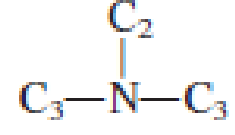Chapter 6, Problem 6.30EP### Organic And Biological Chemistry

7th Edition
STOKER + 1 other
ISBN: 9781305081079

#### Solutions

Chapter
Section### Organic And Biological Chemistry

7th Edition
STOKER + 1 other
ISBN: 9781305081079
Textbook Problem

# How many saturated noncyclic amine constitutional isomers exist that have each of the following generalized formulations? a. C3—NH2 b. C4—NH—C2c. C4—NH—C4

(a)

Interpretation Introduction

Interpretation:

The total number of saturated noncyclic constitutional isomers that exist for the given generalized formula has to be given.

Concept Introduction:

Organic compounds are represented shortly by the molecular formula and structural formula.  Each and every compound has its own molecular formula.  Compounds can have same molecular formula but not same structural formula.

Isomers are the compounds that have same molecular formula but different structural formula.  The main difference lies in the way the atoms are arranged in the structure.  Isomers have different chemical and physical properties even when they have same molecular formula.  This is known as Isomerism.

If there is difference only in the connectivity of the atoms in the molecule, then it is known as constitutional isomerism.  The isomers are known as constitutional isomers.  They will have same molecular formula and same functional group, but they differ in the connectivity between the atoms in the molecule.

Explanation

Given generalized formulation is,

Constitutional isomers are the compounds which have same molecular formula but they differ only in the connectivity of the atoms in the molecule.

The C3 can be arranged in two different ways

(b)

Interpretation Introduction

Interpretation:

The total number of saturated noncyclic constitutional isomers that exist for the given generalized formula has to be given.

Concept Introduction:

Organic compounds are represented shortly by the molecular formula and structural formula.  Each and every compound has its own molecular formula.  Compounds can have same molecular formula but not same structural formula.

Isomers are the compounds that have same molecular formula but different structural formula.  The main difference lies in the way the atoms are arranged in the structure.  Isomers have different chemical and physical properties even when they have same molecular formula.  This is known as Isomerism.

If there is difference only in the connectivity of the atoms in the molecule, then it is known as constitutional isomerism.  The isomers are known as constitutional isomers.  They will have same molecular formula and same functional group, but they differ in the connectivity between the atoms in the molecule.

(c)

Interpretation Introduction

Interpretation:

The total number of saturated noncyclic constitutional isomers that exist for the given generalized formula has to be given.

Concept Introduction:

Organic compounds are represented shortly by the molecular formula and structural formula.  Each and every compound has its own molecular formula.  Compounds can have same molecular formula but not same structural formula.

Isomers are the compounds that have same molecular formula but different structural formula.  The main difference lies in the way the atoms are arranged in the structure.  Isomers have different chemical and physical properties even when they have same molecular formula.  This is known as Isomerism.

If there is difference only in the connectivity of the atoms in the molecule, then it is known as constitutional isomerism.  The isomers are known as constitutional isomers.  They will have same molecular formula and same functional group, but they differ in the connectivity between the atoms in the molecule.

(d)

Interpretation Introduction

Interpretation:

The total number of saturated noncyclic constitutional isomers that exist for the given generalized formula has to be given.

Concept Introduction:

Organic compounds are represented shortly by the molecular formula and structural formula.  Each and every compound has its own molecular formula.  Compounds can have same molecular formula but not same structural formula.

Isomers are the compounds that have same molecular formula but different structural formula.  The main difference lies in the way the atoms are arranged in the structure.  Isomers have different chemical and physical properties even when they have same molecular formula.  This is known as Isomerism.

If there is difference only in the connectivity of the atoms in the molecule, then it is known as constitutional isomerism.  The isomers are known as constitutional isomers.  They will have same molecular formula and same functional group, but they differ in the connectivity between the atoms in the molecule.

### Still sussing out bartleby?

Check out a sample textbook solution.

See a sample solution

#### The Solution to Your Study Problems

Bartleby provides explanations to thousands of textbook problems written by our experts, many with advanced degrees!

Get Started

#### Find more solutions based on key concepts